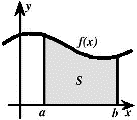## Math in the Middle Institute Partnership## MAT Exam Expository Papers

#### Title

The Polar Coordinate System

#### Date of this Version

7-2008

In partial fulfillment of the requirements for the Master of Arts in Teaching with a Specialization in the Teaching of Middle Level Mathematics in the Department of Mathematics. Jim Lewis, Advisor July 2008

#### Abstract

Representing a position in a two-dimensional plane can be done several ways. It is taught early in Algebra how to represent a point in the Cartesian (or rectangular) plane. In this plane a point is represented by the coordinates (x, y), where x tells the horizontal distance from the origin and y the vertical distance. The polar coordinate system is an alternative to this rectangular system. In this system, instead of a point being represented by (x, y) coordinates, a point is represented by (r, θ) where r represents the length of a straight line from the point to the origin and θ represents the angle that straight line makes with the horizontal axis. The r component is commonly referred to as the radial coordinate and θ as the angular coordinate. Just as in the Cartesian plane, the polar plane has a horizontal axis and an origin. In the polar system the origin is called the pole and the horizontal axis, which is a ray that extends horizontally from the pole to the right, is called the polar axis. An illustration of this can be seen in the figure below: r Polar Coordinate System ~ 2 In the figure, the pole is labeled (0, θ) because the 0 indicates a distance of 0 from the pole, so (0,θ ) will be exactly at the pole regardless of the angle θ . The units of θ can be given in radians or degrees, but generally is given in radians. In this paper we will use both radians and degrees. To translate between radians and degrees, we recall the conversion rules: To convert from radians to degrees, multiply by π 180 To convert from degrees to radians, multiply by 180 π

COinS